Updating search results...

# 674 Results

View
Selected filters:
• GeometryOnly Sharing Permitted
CC BY-NC-ND
Rating
4.5 stars

This lesson unit is intended to help teachers assess how well students are able to visualize two-dimensional cross-sections of representations of three-dimensional objects. In particular, the lesson will help you identify and help students who have difficulties recognizing and drawing two-dimensional cross-sections at different points along a plane of a representation of a three-dimensional object.

Subject:
Geometry
Material Type:
Assessment
Lesson Plan
Provider:
Shell Center for Mathematical Education
Provider Set:
Mathematics Assessment Project (MAP)
04/26/2013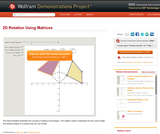Conditional Remix & Share Permitted
CC BY-NC-SA
Rating
5.0 stars

This Demonstration illustrates the concept of rotating a 2D polygon. The rotation matrix is displayed for the current angle. The default polygon is a square that you can modify.

Subject:
Geometry
Material Type:
Activity/Lab
Diagram/Illustration
Homework/Assignment
Interactive
Simulation
Teaching/Learning Strategy
Provider:
Wolfram Research
Provider Set:
Wolfram Demonstrations Project
Author:
Mito Are and Valeria Antohe
09/04/2013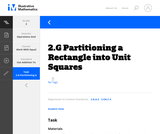Unrestricted Use
CC BY
Rating
0.0 stars

This is a task from the Illustrative Mathematics website that is one part of a complete illustration of the standard to which it is aligned. Each task has at least one solution and some commentary that addresses important aspects of the task and its potential use.

Subject:
Geometry
Material Type:
Activity/Lab
Provider:
Illustrative Mathematics
Provider Set:
Illustrative Mathematics
Author:
Illustrative Mathematics
08/06/2015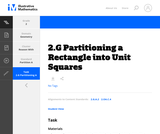Unrestricted Use
CC BY
Rating
0.0 stars

This is a task from the Illustrative Mathematics website that is one part of a complete illustration of the standard to which it is aligned. Each task has at least one solution and some commentary that addresses important aspects of the task and its potential use.

Subject:
Geometry
Material Type:
Activity/Lab
Provider:
Illustrative Mathematics
Provider Set:
Illustrative Mathematics
Author:
Illustrative Mathematics
08/06/2015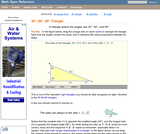Rating
5.0 stars

An interactive applet and associated web page that demonstrate the properties of a 30-60-90 triangle. The applet shows a right triangle that can be resized by dragging any vertex. As it is dragged, the remaining vertices change so that the triangle's angles remain 30 degrees, 60 degrees and 90 degrees The text on the page points out that the sides of a 30-60-90 triangle are always in the ratio of 1 : 2 : root 3 Applet can be enlarged to full screen size for use with a classroom projector. This resource is a component of the Math Open Reference Interactive Geometry textbook project at http://www.mathopenref.com.

Subject:
Geometry
Material Type:
Simulation
Provider:
Math Open Reference
Author:
John Page
02/16/2011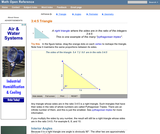Rating
5.0 stars

An interactive applet and associated web page that demonstrate the properties of a 3:4:5 triangle - one of the Pythagorean triples. The applet shows a right triangle that can be resized by dragging any vertex. As it is dragged, the remaining vertices change so that the triangle's side remain in the ration 3:4:5. The text on the page has an example of how the triangle can be used to measure a right angle on even large objects. Applet can be enlarged to full screen size for use with a classroom projector. This resource is a component of the Math Open Reference Interactive Geometry textbook project at http://www.mathopenref.com.

Subject:
Geometry
Material Type:
Simulation
Provider:
Math Open Reference
Author:
John Page
02/16/2011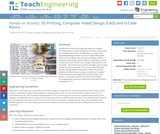Educational Use
Rating
0.0 stars

Students learn how 3D printing, also known as additive manufacturing, is revolutionizing the manufacturing process. First, students learn what considerations to make in the engineering design process to print an object with quality and to scale. Students learn the basic principles of how a computer-aided design (CAD) model is converted to a series of data points then turned into a program that operates the 3D printer. The activity takes students through a step-by-step process on how a computer can control a manufacturing process through defined data points. Within this activity, students also learn how to program using basic G-code to create a wireframe 3D shapes that can be read by a 3D printer or computer numerical control (CNC) machine.

Subject:
Computer Science
Engineering
Geometry
Measurement and Data
Material Type:
Activity/Lab
Provider:
TeachEngineering
Provider Set:
Activities
Author:
Matthew Jourden
05/04/2019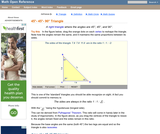Rating
3.0 stars

An interactive applet and associated web page that demonstrate the properties of a 45-45-90 isosceles right triangle. The applet shows a right triangle that can be resized by dragging any vertex. As it is dragged, the remaining vertices change so that the triangle's angles remain 45 degrees, 45 degrees and 90 degrees The text on the page points out that the sides of a 45-45-90 triangle are always in the ratio of 1 : 2 : root 2 Applet can be enlarged to full screen size for use with a classroom projector. This resource is a component of the Math Open Reference Interactive Geometry textbook project at http://www.mathopenref.com.

Subject:
Geometry
Material Type:
Simulation
Provider:
Math Open Reference
Author:
John Page
02/16/2011Conditional Remix & Share Permitted
CC BY-NC-SA
Rating
0.0 stars

Simple angles worksheet for 4th grade math standard

Subject:
Geometry
Material Type:
Lesson Plan
Author:
Ashley Person
06/12/2019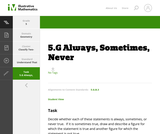Unrestricted Use
CC BY
Rating
0.0 stars

This is a task from the Illustrative Mathematics website that is one part of a complete illustration of the standard to which it is aligned. Each task has at least one solution and some commentary that addresses important aspects of the task and its potential use.

Subject:
Geometry
Material Type:
Activity/Lab
Provider:
Illustrative Mathematics
Provider Set:
Illustrative Mathematics
Author:
Illustrative Mathematics
08/06/2015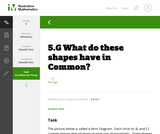Unrestricted Use
CC BY
Rating
0.0 stars

This is a task from the Illustrative Mathematics website that is one part of a complete illustration of the standard to which it is aligned. Each task has at least one solution and some commentary that addresses important aspects of the task and its potential use.

Subject:
Geometry
Material Type:
Activity/Lab
Provider:
Illustrative Mathematics
Provider Set:
Illustrative Mathematics
Author:
Illustrative Mathematics
08/06/2015Unrestricted Use
CC BY
Rating
0.0 stars

Family facing 6th Grade math unit focusing on area and surface area.

Subject:
Geometry
Material Type:
Unit of Study
Provider:
Illustrative Mathematics
05/11/2020Unrestricted Use
CC BY
Rating
0.0 stars

In this unit, students learn to find areas of polygons by decomposing, rearranging, and composing shapes. They learn to understand and use the terms “base” and “height,” and find areas of parallelograms and triangles. Students approximate areas of non-polygonal regions by polygonal regions. They represent polyhedra with nets and find their surface areas.

Subject:
Geometry
Material Type:
Unit of Study
Provider:
Illustrative Mathematics
05/11/2020Unrestricted Use
CC BY
Rating
0.0 stars

Student facing 6th Grade math unit focusing on area and surface area.

Subject:
Geometry
Material Type:
Unit of Study
Provider:
Illustrative Mathematics
05/11/2020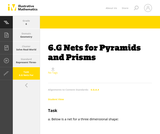Unrestricted Use
CC BY
Rating
0.0 stars

This is a task from the Illustrative Mathematics website that is one part of a complete illustration of the standard to which it is aligned. Each task has at least one solution and some commentary that addresses important aspects of the task and its potential use.

Subject:
Geometry
Material Type:
Activity/Lab
Provider:
Illustrative Mathematics
Provider Set:
Illustrative Mathematics
Author:
Illustrative Mathematics
08/06/2015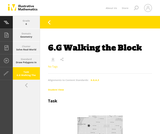Unrestricted Use
CC BY
Rating
0.0 stars

This is a task from the Illustrative Mathematics website that is one part of a complete illustration of the standard to which it is aligned. Each task has at least one solution and some commentary that addresses important aspects of the task and its potential use.

Subject:
Geometry
Material Type:
Activity/Lab
Provider:
Illustrative Mathematics
Provider Set:
Illustrative Mathematics
Author:
Illustrative Mathematics
08/06/2015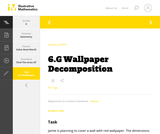Unrestricted Use
CC BY
Rating
0.0 stars

This is a task from the Illustrative Mathematics website that is one part of a complete illustration of the standard to which it is aligned. Each task has at least one solution and some commentary that addresses important aspects of the task and its potential use.

Subject:
Geometry
Material Type:
Activity/Lab
Provider:
Illustrative Mathematics
Provider Set:
Illustrative Mathematics
Author:
Illustrative Mathematics
08/06/2015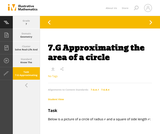Unrestricted Use
CC BY
Rating
0.0 stars

This is a task from the Illustrative Mathematics website that is one part of a complete illustration of the standard to which it is aligned. Each task has at least one solution and some commentary that addresses important aspects of the task and its potential use.

Subject:
Geometry
Material Type:
Activity/Lab
Provider:
Illustrative Mathematics
Provider Set:
Illustrative Mathematics
Author:
Illustrative Mathematics
08/06/2015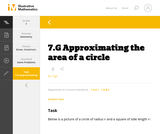Unrestricted Use
CC BY
Rating
0.0 stars

This is a task from the Illustrative Mathematics website that is one part of a complete illustration of the standard to which it is aligned. Each task has at least one solution and some commentary that addresses important aspects of the task and its potential use.

Subject:
Geometry
Material Type:
Activity/Lab
Provider:
Illustrative Mathematics
Provider Set:
Illustrative Mathematics
Author:
Illustrative Mathematics
08/06/2015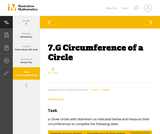Unrestricted Use
CC BY
Rating
0.0 stars

This is a task from the Illustrative Mathematics website that is one part of a complete illustration of the standard to which it is aligned. Each task has at least one solution and some commentary that addresses important aspects of the task and its potential use.

Subject:
Geometry
Material Type:
Activity/Lab
Provider:
Illustrative Mathematics
Provider Set:
Illustrative Mathematics
Author:
Illustrative Mathematics